# Math in Focus Grade 6 Chapter 12 Answer Key Surface Area and Volume of Solids

This handy Math in Focus Grade 6 Workbook Answer Key Chapter 12 Surface Area and Volume of Solids detailed solutions for the textbook questions.

## Math in Focus Grade 6 Course 1 B Chapter 12 Answer Key Surface Area and Volume of Solids

### Math in Focus Grade 6 Chapter 12 Quick Check Answer Key

Name each, prism. In each prism identify a base, a face, an edge, and a vertex.

Question 1.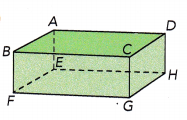It is a Rectangular prism.
It has 2 bases, 6 faces, 12 edges and 8 vertices.

Question 2.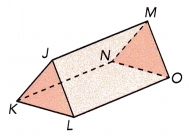It is a triangular prism.
It has 2 bases, 5 faces, 9 edges and 6 vertices.

Question 3.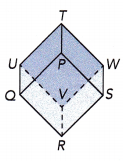It is a square prism.
It has 2 bases, 6 faces, 12 edges and 8 vertices.

Find the area of each figure.

Question 4.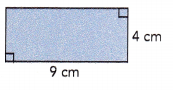Area =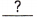=cm2
The given rectangle is 4cm wide and 9cm long.
The formula for finding the area of rectangle is length×breadth.
The area for the given rectangle is 4×9=36 square centimetre.

Question 5.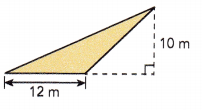Area = $$\frac{1}{2}$$ •=m2

The given trinagle measures 12m wide and 10m long.
The formula to find the area of the given triangle is $$\frac{1}{2}$$×base×height
Therefore, the area of the given triangle will be $$\frac{1}{2}$$×12×10 = 60 square metre.
Question 6.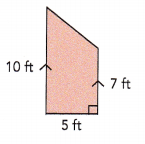Area = $$\frac{1}{2}$$ •• (+)
= $$\frac{1}{2}$$ •=ft2
The given quadrilateral has two sides of 10 ft and 7 ft with 5ft base.
Area of the trapezium = $$\frac{1}{2}$$ × height × (sum of parallel sides)
The area will be $$\frac{1}{2}$$×5×(10+7) = $$\frac{1}{2}$$×5×12
Therefore, the area will be 5×6=30 square ft.

Question 7.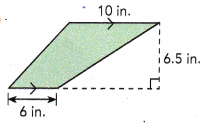Area = $$\frac{1}{2}$$ •• (+)
= $$\frac{1}{2}$$ •=in.2
The formula to find the area of trapezium = $$\frac{1}{2}$$ × height × (sum of parallel sides)
Area = $$\frac{1}{2}$$ × 6.5 × (10+6)
= $$\frac{1}{2}$$ × 6.5 × 16
= 6.5×8
= 52 square in.

Find the volume of each solid.

Question 8.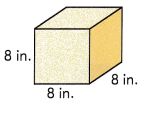Volume ==in.3
Volume of the cube = length×width×height
The given cube measures 8 inches in length.
The volume of the given cube wil be 8×8×8=64 cubic.inches

Question 9.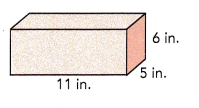Volume ==in.3
The given cuboid has length of 11 in, width of 5 in and height of 6 in.
Volume of the cuboid = length×width×height
The volume of the given cuboid is 11×5×6=330 cubic. inches.

Question 10.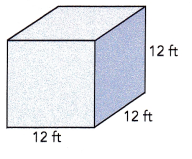Volume ==ft3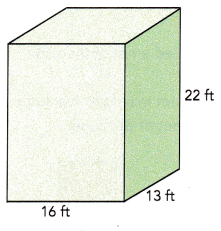Volume ==ft3